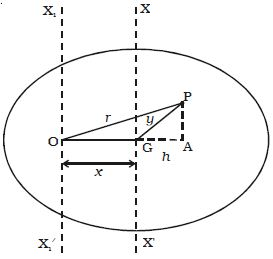# Theorems of Moment of Inertia

Theorems of Moment of Inertia:

(i) Parallel axes theorem:

The moment of inertia of a body about any axis is equal to the sum of its moment of inertia about a parallel axis through its centre of gravity and the product of the mass of the body and the square of the distance between the two axes.Fig: Parallel axes theorem

(ii) Perpendicular axes theorem

The moment of inertia of a plane laminar body about an axis perpendicular to the plane is equal to the sum of the moments of inertia about two mutually perpendicular axes in the plane of the lamina such that the three mutually perpendicular axes have a common point of intersection.Fig: Perpendicular axes theorem